# Nitrogen trifluoride (NF3) lewis dot structure, molecular geometry, polar or non-polar, hybridization

Home  > Chemistry Article > NF3 lewis structure and its molecular geometryIn this article, we will discuss NF3 lewis dot structure, molecular geometry or VSEPR shape, bond angle, hybridization, etc. We will also discuss is NF3 polar or non-polar?

NF3 is used to make chemicals or also used as a component of rocket fuels. It is a strong greenhouse gas and it has the potential to reduce global warming greater than co2. It is also used for making chemical lasers like deuterium fluoride and hydrogen fluoride laser.

Properties of Nitrogen trifluoride

• It has a molar mass of 71.00 g/mol.
• NF3 boiling point is −129.06 °C and melting point is −207.15 °C.
• It has a low dipole moment.
• The density of NF3 is 3.003 kg/m3
• Its refractive index is 1.0004.
• It is Corrosive to tissue.
 Name of Molecule Nitrogen trifluoride Chemical formula NF3 Molecular geometry of NF3 Trigonal pyramid Electron geometry of NF3 Tetrahedral Hybridization Sp³ Bond angle 101.9º Total Valence electron for NF3 26 The formal charge of NF3 0
Page Contents

## How to draw NF3 lewis structure?

NF3 lewis structure has 3 fluorine and 1 nitrogen atom connected with three single bonds. This structure is very similar to NCl3 and NH3. There is a total of 10 lone pairs and 3 bonded pairs present in the NF3 lewis structure.

Let’s see how to draw lewis dot structure for NF3 with easy steps.

## Simple steps for drawing the NF3 lewis dot structure

1. Count total valence electron in NF3

In the first step, we have to calculate the total number of valence electrons present in the NF3 molecule. As nitrogen present at 15th group in periodic table and Fluorine in group 17th.

⇒ Total valence electron in Nitrogen = 5

⇒ Total valence electron in Fluorine = 7

∴ Total valence electron available for drawing the NF3 lewis structure = 5 + 7*3 = 26 valence electrons     [∴NF3 has three fluorine atom and one nitrogen]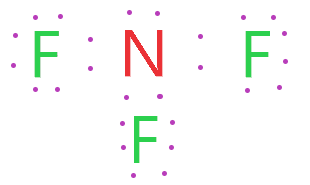2. Find the least electronegative atom and placed it at center

After finding the total valence electron next step is to determine which atom has less electronegativity.

For this remember electronegativity increase from left to right in the periodic table. Clearly, nitrogen is in the left position to fluorine in the periodic table.

Nitrogen is the least electronegative atom in the NF3 molecule. So, placed it at the center, and fluorine spaced evenly around it.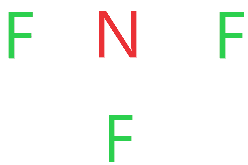3. Connect nitrogen and fluorine with a single bond

In this step, we start drawing NF3 skeletal structure by bonding together Nitrogen and Fluorine with a single bond.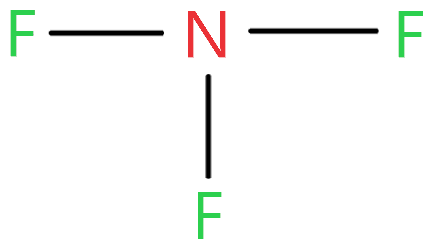Now look at this structure and count how many electrons we used till now and how many are left.

As three single bonds are used in the above structure that contains 6 electrons and we have a total of 26 valence electrons are available for NF3 lewis structure.

∴ (26 – 6) = 20 valence electrons

So, we are left with 20 valence electrons.

4. Placed remaining valence electrons starting from outer atom first

In this step, we have to place the remaining valence electrons starting from the more electronegative atom or from the outer atoms.

So, fluorine is more electronegative than nitrogen and it needs 8 electrons to complete its octet rule.

Fluorine already shares 2 electrons with the help of a single bond so it only needs 6 more electrons to complete its octet rule.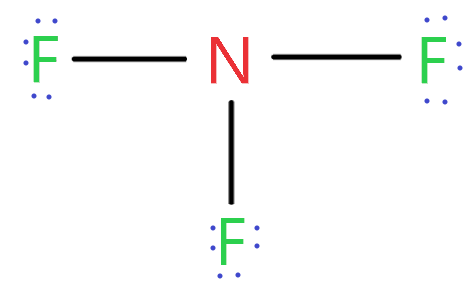As you see in the above structure, each fluorine use 6 electrons to complete its octet. Now find how many valence electrons are left.

Three fluorine in the above structure used 18 electrons from a total of 20 remaining valence electrons.

∴ (20 – 18) = 2 valence electrons

So, we left with 2 electrons.

Placed these 2 electrons around the central atom(Nitrogen).

5. Complete central atom octet  and use covalent bond if necessary

After placing two electrons around the central atom, nitrogen also completes its octet rule because it already shares three single bonds with fluorine which contain 6 electrons.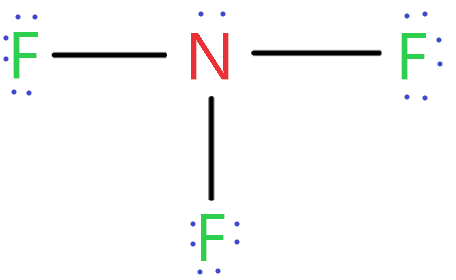### NF3 lewis structure

As you see in this NF3 lewis dot structure, nitrogen and fluorine already complete their octet rule so no need to make any covalent bond.

We got our correct Nitrogen trifluoride lewis structure.

## What are the electron and molecular geometry of NF3?

The molecular geometry of NF3 is a trigonal pyramid and its electron geometry is tetrahedral because nitrogen has Sp³ hybridization with 5 valence electrons in its valence shell and it makes three bond pairs, one with each fluorine atom.

And one lone pair is present on the nitrogen atom that tries to repel the bonded pairs of electrons, as a result, the adjacent atoms push apart giving its geometry trigonal pyramid.As you see in the above structure, the lone pair on the nitrogen atom repel the adjacent electron pairs, as a result, the two fluorine atom in equatorial position pushes far apart giving the NF3 molecular geometry same as a trigonal pyramid.

Let’s see how to find the molecular and electron geometry of NF3 this step by step.

### 1. Find the Number of lone pairs present on the central atom of the NF3 lewis structure

To find the number of lone pairs, you can use the Nitrogen trifluoride lewis structure. So, according to it, NF3 central atom contains 1 lone pair.

Or find lone pair by using the formula.

∴ L.P = (V.E. – N.A.)/2

where L.P. = Lone pair on the central atom

V.E. = valence electron of that central atom

N.A. = Number of atoms attached to that central atom

∴ So, Nitrogen is the central atom, it has 5 valence electrons and the number of atoms attached to nitrogen is 3

So, L.P. = (5 – 3)/2

= 1

Hence, lone pair on the central atom nitrogen is 1.

### 2. Find hybridization number of NF3

Use the formula to find the hybridization of NF3.

∴ H = N.A. + L.P.

where H = hybridization number

N.A. = Number of atoms attached to the central atom

L.P. = lone pairs on that central atom

So, the central atom (nitrogen) attached to 3 fluorine atoms and it has 1 lone pair.

So, H = 3 + 1

= 4

Hence the hybridization number of the NF3 central atom is 4 which means it has Sp³ hybridization.

### 3. Use VSEPR theory or AXN method to determine NF3 molecular shape

According to Valence shell electron pair repulsion theory if the molecule contains Sp³ hybridization and 1 lone pair of electrons, then the shape of the molecule is trigonal pyramidal.### NF3 molecular geometry or Shape

You can use the AXN method also to determine the molecular geometry or electron geometry of NF3.

• A represents the central atom.
• X represents the bonded atoms to the central atom.
• N represents the lone pairs on the central atom

So, nitrogen is the central atom that has 1 lone pair and 3 bonded pair electrons according to the NF3 lewis dot structure.

Hence the formula of NF3 becomes AX3N1

So, according to the VSEPR chart, if the molecule has the formula of AX3N1, it has a molecular shape of trigonal pyramid and electron geometry of tetrahedral.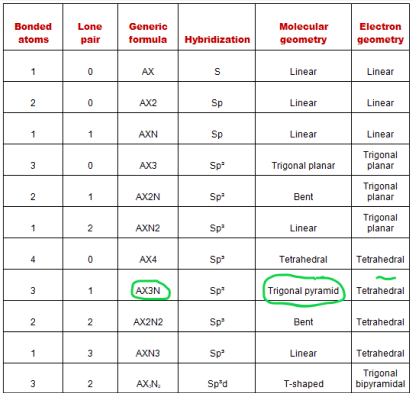The bond angle of Nitrogen trifluoride (NF3) is 101.9°.

## Nitrogen trifluoride polarity: is NF3 polar or nonpolar

NF3 is polar because of its asymmetric shape and electronegativity difference between nitrogen and fluorine which cause non-zero dipole moment.

For knowing, is NF3 polar or non-polar? we will discuss three factors 1. electronegativity 2. dipole moment 3. Geometrical or molecular shape.

## Three factors that indicate the polarity of NF3

1. Electronegativity:

The difference between the electronegativity of Nitrogen and fluorine is directly proportional to the Polarity of the NF3 molecule.

The electronegativity of the fluorine atom is 3.98 and for the nitrogen atom, its value is 3.04.

So, being more electronegativity of fluorine help it to attract more bonded electron than nitrogen. Therefore fluorine gains a partial negative charge and nitrogen gains a partial positive charge.

Positive and negative charges cause non-uniform charge distribution around the NF3 structure. Hence it also causes NF3 to become polar in nature.

Also, the electronegativity difference of fluorine and nitrogen atom is greater than 0.5 which ensures that NF3 is highly polar in nature.

2. Dipole moment

The net dipole moment of the NF3 molecule is 0.24 D. Dipole moment measure the strength of polarity.

The larger the electronegativity differences of atoms greater is the polarity and dipole moment.

The dipole of the NF3 molecule originates in the downward and upward direction because of the lone pair present in Nitrogen. Hence it causes a non-zero dipole moment which causes the polar nature of NF3.

⇒ Dipole moment formula =  charge on the atoms * the distance between them

∴ D = Q × R

3. Geometrical or molecular shape

The geometrical shape is also a big factor to determine is NF3 polar or non-polar.

As we know NF3 molecular geometry is trigonal pyramidal which is asymmetric and when the shape of the molecule is asymmetric or distorted it doesn’t cancel the dipole moment across the molecule. Hence cause polar nature of the molecule

All these factors are enough to understand whether NF3 is polar or non-polar.

## What is the formal charge in NF3 lewis dot structure and how to calculate it?

To calculate the formal charge in NF3. Use this equation:

⇒ Formal charge = (Valence electrons – unbonded electrons – 1/2 bonded electrons)

⇒ We will calculate the formal charge on Nitrogen which is the central atom in the Nitrogen trifluoride lewis diagram.

⇒ Valence electron of nitrogen = 5

⇒ Non-bonding electrons = 2

⇒ Bonding electrons = 6

∴ 5 – 2 – 6/2

= 0 is the formal charge on Nitrogen.

## FAQ

### Why bond angle in NF3 less than that in NH3?

The bond angle is inversely proportional to electronegativity. So, in the case of NF3, fluorine is more electronegative than nitrogen hence it attracts more electrons. Hence repulsion between the bond of nitrogen decreased and it makes NF3 bond angle lower.

In the case of NH3, nitrogen is more electronegative than hydrogen. Hence it attracts more electrons and repulsion between the bond of nitrogen increased. Hence it causes the bond angle of NH3 higher than NF3.

∴ l.p.-l.p. > l.p-b.p >b.p-b.p.

where l.p. = lone pairs

b.p. = bond pairs

### How many lone pairs and bond pairs are present around Nitrogen in the NF3 lewis structure?

Nitrogen contains 1 lone pair and 3 bonded pair which is attached with fluorine atoms according to the NF3 lewis structure.

### why NF3 is less polar than NH3?

N-F bond is more polar than N-H but NF3 is less polar than NH3. In NF3, polarity is attracted towards fluorine because of more electronegativity. Hence some of the dipole moments are cancelled out because polarity is attracted opposite to the centre atom.

The dipole moment of NF3 is 0.24 D.

In NH3, nitrogen is more electronegative than hydrogen hence dipole moment will add up because polarity is attracted towards the centre from hydrogen to nitrogen.

The dipole moment of NH3 is 1.47 D.

Hence greater is the dipole moment of the Molecule more is the polarity of that molecule.

Hence NH3 is more polar than NF3.

## Summary

• The total valence electron available for the NF3 lewis structure is 26.
• Hybridization of NF3 is Sp³.
• NF3 is polar in nature.
• The molecular geometry or shape of NF3 is a trigonal pyramid and its electron geometry is tetrahedral.
• NF3 lewis dot structure contains 1 lone pair and 3 bonded pairs.
##### Subscribe to Blog via Email

Join 2 other subscribers

Share it...